Courses

# Chebyshev Filter Design Electrical Engineering (EE) Notes | EduRev

## Electrical Engineering (EE) : Chebyshev Filter Design Electrical Engineering (EE) Notes | EduRev

The document Chebyshev Filter Design Electrical Engineering (EE) Notes | EduRev is a part of the Electrical Engineering (EE) Course Digital Signal Processing.
All you need of Electrical Engineering (EE) at this link: Electrical Engineering (EE)

Chebyshev Filter Design

Defined as Hc(S) Hc(-S) =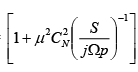μ= measure of allowable deviation in the pass band.

CN(x) = Cos(NCos-1(x)) is the Nth order polynomial.

Let x = Cos θ

CN(x) = Cos(Nθ)

C0(x) = 1

C1(x) = Cosθ =x

C2(x) = Cos2θ = 2 Cos2θ -1 = 2x2-1

C3(x) = Cos3 θ = 4 Cos3 θ -3 Cos θ = 4x3-3x     etc..

 N CN(x) 0 1 1 x 2 2x2-1 3 4x3-3x 4 8x4- 8x2 +1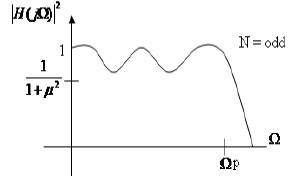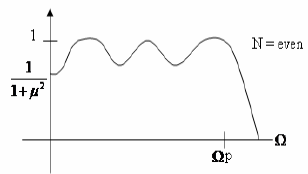Two features of Chebyshev poly are important for the filter design

1.| CN (x)| ≤ 1    for|x| ≤ 1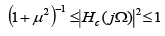for  0 ≤ Ω ≤ Ωp

Transfer function lies in the range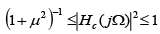for  0 ≤ Ω ≤Ωp Whereas the frequency value important for the design of the Butterworth filter was the Ωc the relevant frequency for the Chebyshev filter is the edge of pass band Ωp.

2. |X| >>1|CN,l Increases as the Nth power of x. this indicates that for Ω>>Ωp, the magnitude response decreases as Ω-N, or -6N dB Octane. This is identical to Butterworth filter.

Now the ellipse is defined by major & minor axis.

Define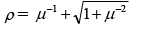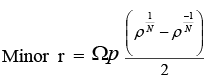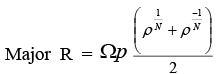N = Order of filter.

SP = r Cosθ+j R Sinθ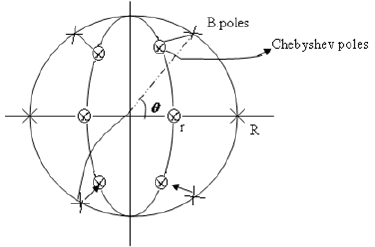Ex:

Pass band:

-1<|H ( jΩ)|2dB ≤ -60   for 0 ≤ Ω ≤ 1404π

Stop band:

|H ( jΩ)|2dB < -60   for Ω ≥ 8268π

Value of μ is determined from the pass band

10 log(1 +μ 2 )-1 > -1dB   -1dB = 0.794

μ< [ 100.1-1 ]1/2 = 0.508

μ= 0.508

Value of N is determined from stop band inequality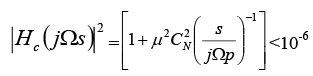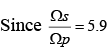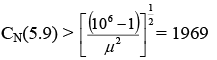Evaluating

C3(5.9) = 804 C4(5.9)  = 9416 therefore N = 4 is sufficient.

Since this last inequality is easily satisfied with N=4 the value of μ can be reduced to as small as 0.11, to decrease pass band ripple while satisfying the stop band. The value  μ =0.4 provides a margin in both the pass band and stop band. We proceed with the design with μ =0.508 to show the 1dB ripple in the pass band.

Axes of Ellipse: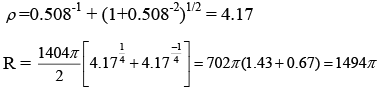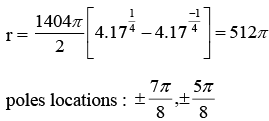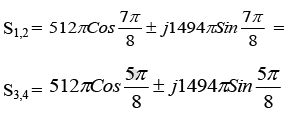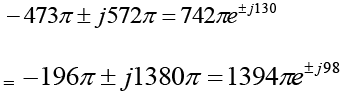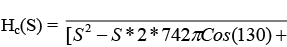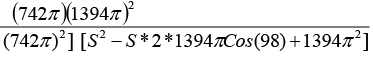• Chebyshev filter poles are closer to the jΩ axis, therefore filter response exhibits a  ripple in the pass band. There is a peak in the pass band for each pole in the filter, located approximately at the ordinate value of the pole.
• Exhibits a smaller transition region to reach the desired attenuation in the stop band, when compared to Butterworth filter.
• Phase response is similar.
• Because of proximity of Chebyshev filter poles to j Ω axis, small errors in their locations, caused by numerical round off in the computations, can results in significant changes in the magnitude response. Choosing the smaller value of μ will provide some margin for keeping the ripples within the pass band specification. However, too small a value for  μ may require an increase in the filter order.
• It is reasonable to expect that if relevant zeros were included in the system function, a lower order filter can be found to satisfy the specification. These relevant zeros could serve to achieve additional attenuation in the stop band. The elliptic filter does exactly this.
Offer running on EduRev: Apply code STAYHOME200 to get INR 200 off on our premium plan EduRev Infinity!

,

,

,

,

,

,

,

,

,

,

,

,

,

,

,

,

,

,

,

,

,

;